Question

8) A five-year coupon bond with a face value of \$1000 is sold in the primary market. The interest rate is 8%. Immediately after receiving the second coupon payment the bondholder sells the bond into the secondary market. At the time of resale the interest rate has dropped to 6%. What is the resale price of the bond?
a) 948.46
b) 986.29
c) 1025.31
d) 1053.46

We need at least 10 more requests to produce the answer.

0 / 10 have requested this problem solution

The more requests, the faster the answer.

All students who have requested the answer will be notified once they are available.

#### Earn Coins

Coins can be redeemed for fabulous gifts.

Similar Homework Help Questions
• ### Suppose you purchase 5-year annual coupon bond in the primary market. The face value of the...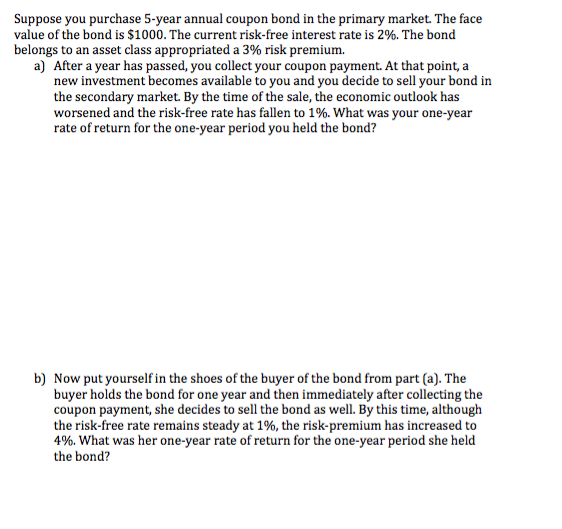Suppose you purchase 5-year annual coupon bond in the primary market. The face value of the bond is \$1000. The current risk-free interest rate is 2%. The bond belongs to an asset class appropriated a 3% risk premium. a) After a year has passed, you collect your coupon payment. At that point, a new investment becomes available to you and you decide to sell your bond in the secondary market. By the time of the sale, the economic outlook has...

• ### a) (5 points) Calculate the price of a \$1000 face value five year coupon bond when...

a) (5 points) Calculate the price of a \$1000 face value five year coupon bond when the yield to maturity is 5%, and the coupon rate is 6%. b) (5 points) Now suppose that the yield to maturity rises to 7%. Calculate the new price of this coupon bond. c) (5 points) Suppose you purchased the bond at it original price (yield to maturity = 5%) held it for one year (collected one coupon payment) and sold it at the...

• ### 4) Suppose there is a 3-year bond with a \$1000 face value, 30% annual coupon payments...

4) Suppose there is a 3-year bond with a \$1000 face value, 30% annual coupon payments and a 20% annual yield to maturity. a) Without any calculation, briefly explain whether this bond will be selling a premium or a discount. b) Calculate the price of this bond. c) Calculate the duration of this bond. d) Suppose the interest rates in the economy rise by 5 percentage points immediately after someone bought this bond. Show a calculation using duration for what...

• ### 4) Suppose there is a 3-year bond with a \$1000 face value, 30% annual coupon payments...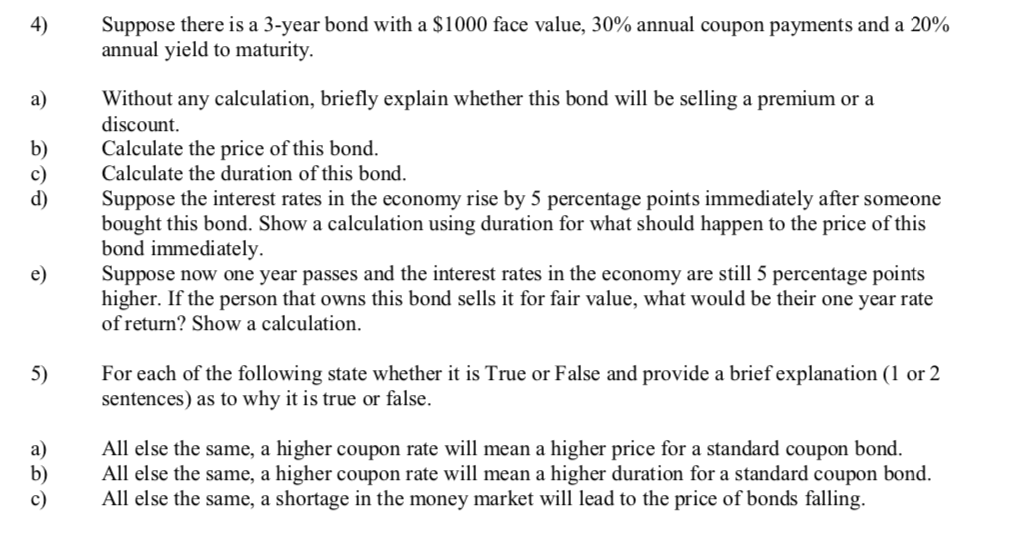4) Suppose there is a 3-year bond with a \$1000 face value, 30% annual coupon payments and a 20% annual yield to maturity. a) Without any calculation, briefly explain whether this bond will be selling a premium or a discount b) Calculate the price of this bond. c Calculate the duration of this bond. d) Suppose the interest rates in the economy rise by 5 percentage points immediately after someone bought this bond. Show a calculation using duration for what...

• ### (1 point) A 9-year bond with a face value of 1000 dollars is redeemable at par,...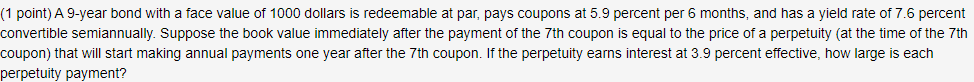(1 point) A 9-year bond with a face value of 1000 dollars is redeemable at par, pays coupons at 5.9 percent per 6 months, and has a yield rate of 7.6 percent convertible semiannually. Suppose the book value immediately after the payment of the 7th coupon is equal to the price of a perpetuity (at the time of the 7th coupon) that will start making annual payments one year after the 7th coupon. If the perpetuity earns interest at 3.9...

• ### Suppose there is a 3-year bond with a \$1000 face value, 30% annual coupon payments and...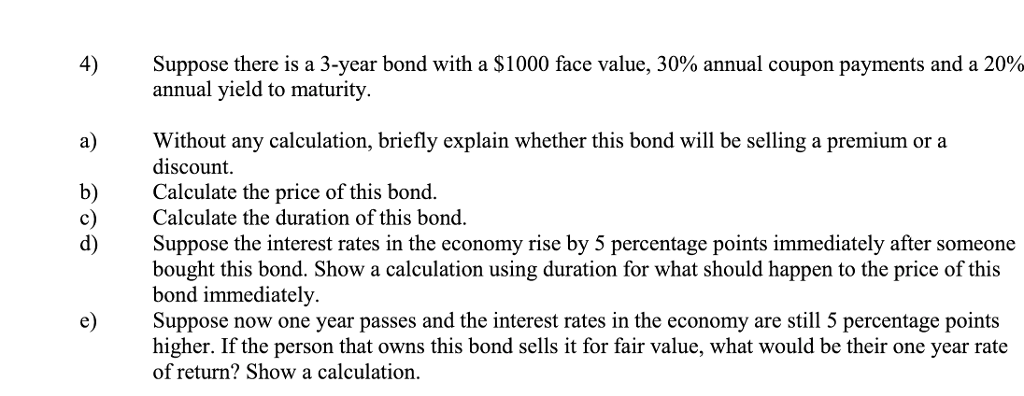Suppose there is a 3-year bond with a \$1000 face value, 30% annual coupon payments and a 20% annual yield to maturity. 4) a Without any calculation, briefly explain whether this bond will be selling a premium or a discount. b) Calculate the price of this bond. c) Calculate the duration of this bond. d) Suppose the interest rates in the economy rise by 5 percentage points immediately after someone bought this bond. Show a calculation using duration for what...

• ### Suppose there is a 3-year bond with a \$1000 face value, 30% annual coupon payments and...

Suppose there is a 3-year bond with a \$1000 face value, 30% annual coupon payments and a 20% annual yield to maturity. a) Without any calculation, briefly explain whether this bond will be selling a premium or a discount. b) Calculate the price of this bond. c) Calculate the duration of this bond. d) Suppose the interest rates in the economy rise by 5 percentage points immediately after someone bought this bond. Show a calculation using duration for what should...

• ### 4) Suppose there is a 3-year bond with a \$1000 face value, 30% annual coupon payments...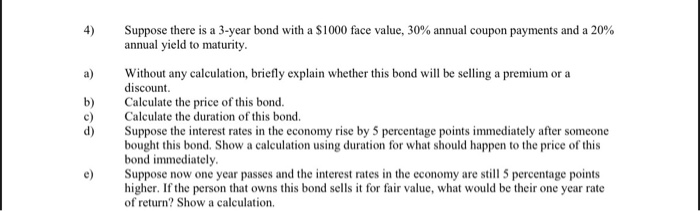4) Suppose there is a 3-year bond with a \$1000 face value, 30% annual coupon payments and a 20% annual yield to maturity a) Without any calculation, briefly explain whether this bond will be selling a premium or a discount Calculate the price of this bond Calculate the duration of this bond Suppose the interest rates in the economy rise by 5 percentage points immediately after someone bought this bond. Show a calculation using duration for what should happen to...

• ### 4) Suppose there is a 3-year bond with a \$1000 face value, 30% annual coupon payments...

4) Suppose there is a 3-year bond with a \$1000 face value, 30% annual coupon payments and a 20% annual yield to maturity. a) Without any calculation, briefly explain whether this bond will be selling a premium or a discount. b) Calculate the price of this bond. c) Calculate the duration of this bond. d) Suppose the interest rates in the economy rise by 5 percentage points immediately after someone bought this bond. Show a calculation using duration for what...

• ### 1. You own a 20-year, \$1000 face value bond paying 8% coupon annually. If market price...

1. You own a 20-year, \$1000 face value bond paying 8% coupon annually. If market price of the bond is 1000, what should be the Yield to Maturity of the bond? You also own a 20-year, \$1000 face value bond paying 8% coupon annually. What should be the market price of the bond so that its Yield to Maturity is exactly 10%?